# Connect Visual to Algebraic Representation of Pythagorean Theorem

## Tapping It Up a Notch: Pythagorean Theorem – Part 3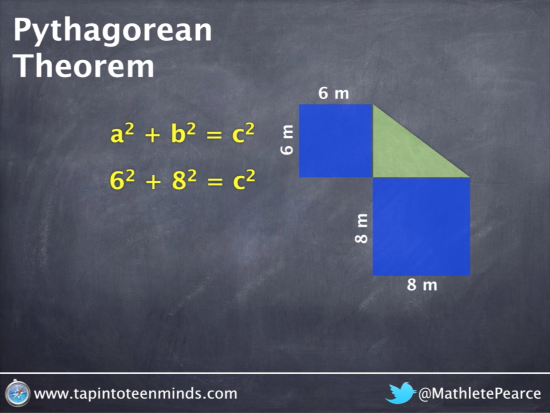In the first part, we used an inquiry/discovery approach to help students visualize how Pythagorean Theorem works, then followed that with a visualization of the general proof / derivation of the formula to show how we can find the hypotenuse of a right-angle triangle when given the lengths of the two legs.

In this post, we will now make a connection between the visual representation of the Pythagorean Theorem with the algebraic representation to show students that the intent of algebra is not to confuse, but rather to make calculations more easily when patterns have been generalized as they have here.

## Using Pythagorean Theorem to Find the Length of the Hypotenuse

### Connecting the Visual Representation and Algebraic Representation

In this video, we now attempt to make a connection between the visual representation and algebraic representation for a specific right-angle triangle. Ensuring students have already watched the first video and second video from part one and part two of this series is important to maximize the effectiveness.

## Summary of Pythagorean Theorem Video

We now start with variables representing the side lengths of 6 metres and 8 metres in an attempt to find the length of the hypotenuse.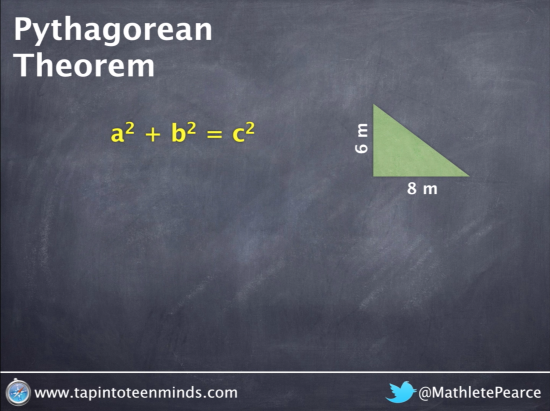### Connect Squaring Side-Lengths to Area Visually and Algebraically

We show that squaring the side lengths on the right-angle triangle will yield an area since we are multiplying length by width (or side by side, in this case).### Evaluate Squaring The Length of the Legs

Squaring 6 m and 8 m yields areas of 36 m^2 and 64 m^2, respectively. This is shown algebraically as well as visually: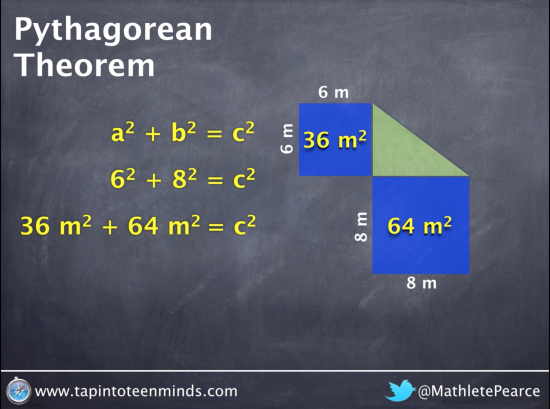### Show The Sum of the Squares of the Leg Lengths

We now calculate the sum of the two squares, 36 m^2 plus 64 m^2 which gives the result of 100 m^2. We show what this looks like in the formula as well as visually.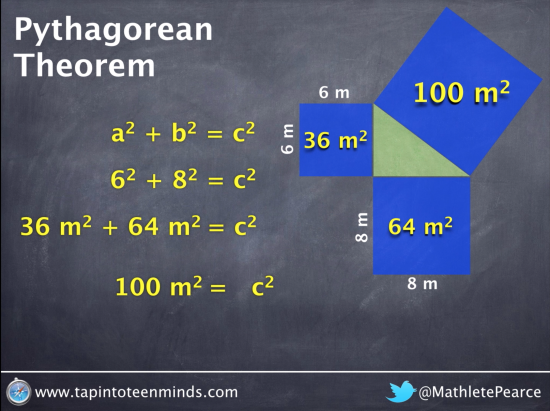### Square-Root to Determine the Side Lengths

In order to determine the side length of the hypotenuse square, we must now square-root. This is a great place to discuss opposite operations and make the connection between square-rooting 100 m^2 as well as c^2.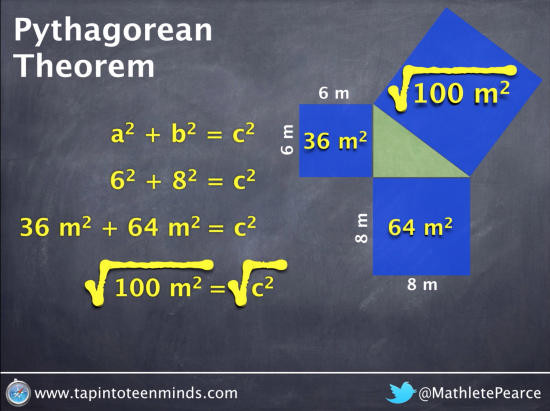### Final Result of 10 m for the Length of the Hypotenuse

Square-rooting both sides of the equation as well as the visual representation will give the result of 10 m for the length of the hypotenuse.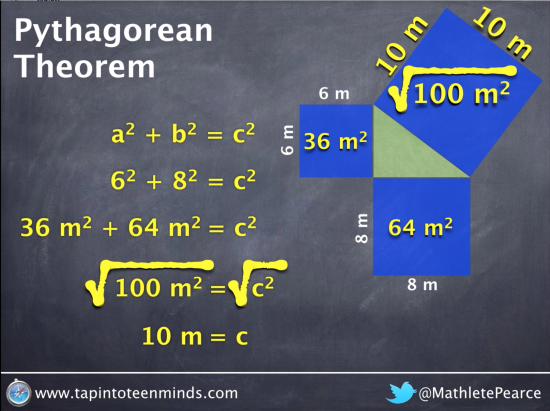What are your thoughts? How can we improve these videos to better assist students understanding how the algebraic representation of Pythagorean Theorem works? Leave a comment below!

## Other Related Pythagorean Theorem Posts:

[postshortcode the_query=”post_type=post&p=14140″]

[postshortcode the_query=”post_type=post&p=14156″]

[threeactshortcode the_query=”post_type=realworldmath&p=3760″]

## WANT TO LEARN HOW TO TEACH THROUGH TASK?

Download our Complete Guide to successfully implementing our Make Math Moments 3-Part Framework in your math class!## Share With Your Learning Community:I’m Kyle Pearce and I am a former high school math teacher. I’m now the K-12 Mathematics Consultant with the Greater Essex County District School Board, where I uncover creative ways to spark curiosity and fuel sense making in mathematics. Read more.